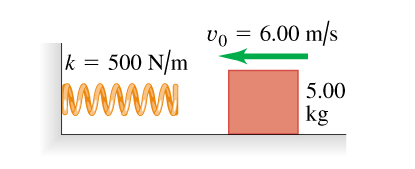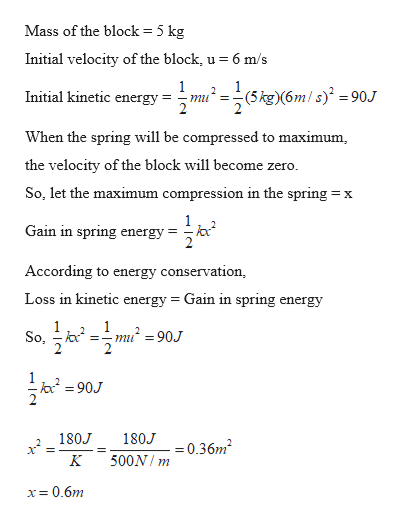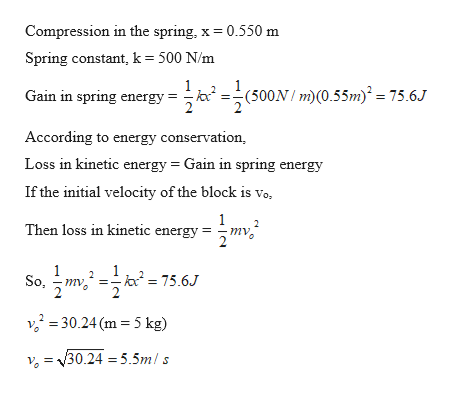# vo 6.00 m/sk = 500 N/m5.00kg

Question
333 views

A 5.00-kg block is moving at 6.00 m/s along a frictionless, horizontal surface toward a spring with force constant k=500 N/m that is attached to a wall (the figure (Figure 1)). The spring has negligible mass.

Find the maximum distance the spring will be compressed.
If the spring is to compress by no more than 0.550 m , what should be the maximum value of v0?help_outlineImage Transcriptionclosevo 6.00 m/s k = 500 N/m 5.00 kg fullscreen
check_circle

Step 1

Given:

Mass of the block = 5 kg

Velocity of the block = 6 m/s

Spring constant = 500 N/m

Step 2

Calculating the maximum compression in the spring:help_outlineImage TranscriptioncloseMass of the block = 5 kg Initial velocity of the block, u = 6 m/s 1 Initial kinetic energy :(m/s =90.J (5 kg)(6m miu When the spring will be compressed to maximum the velocity of the block will become zero So, let the maximum compression in the spring: X Gain in spring energy According to energy conservation, Loss in kinetic energy = Gain in spring energy 1 So, 1 mu=90J 2 2 90J 180J 180J 0.36m2 500N m x= 0.6m fullscreen
Step 3

Finding the required velo...help_outlineImage TranscriptioncloseCompression in the spring, x 0.550 m Spring constant, k= 500 N/m (500m)(0.55m = 75.6J Gain in spring energy According to energy conservation Loss in kinetic energy Gain in spring energy If the initial velocity of the block is vo, 1 mv Then loss in kinetic energy So 30.24 (m 5 kg) v=30.24 =5.5mls fullscreen

### Want to see the full answer?

See Solution

#### Want to see this answer and more?

Solutions are written by subject experts who are available 24/7. Questions are typically answered within 1 hour.*

See Solution
*Response times may vary by subject and question.
Tagged in

### Newtons Laws of Motion